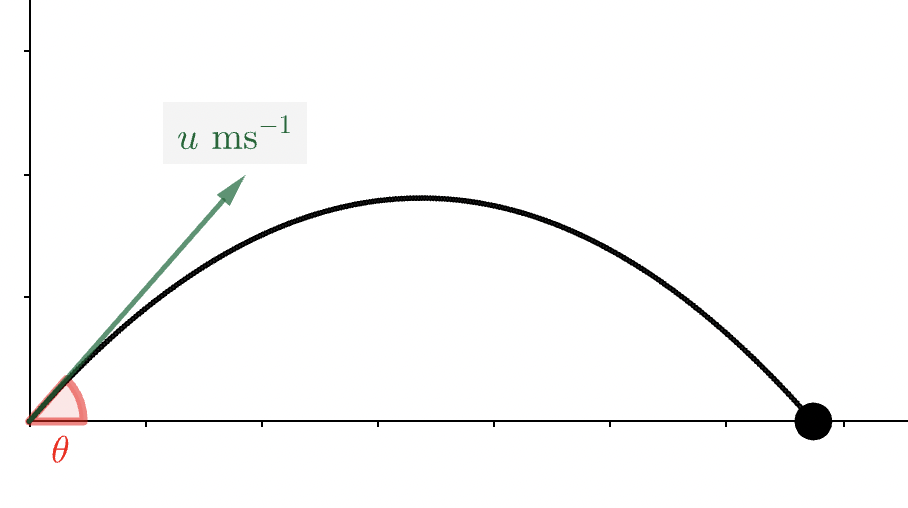Overview:

# Part 4: Projectile motion formulaeIf a particle is projected from point $$(0,0)$$ with initial velocity $$u$$ at an angle $$\theta$$ above the horizontal, then (assuming it moves freely under gravity) we can deduce the following:

• Range on horizontal plane = $$\dfrac{u^{2}\text{sin}\left(2\theta\right)}{g}$$
• Time of flight = $$\dfrac{2u\text{sin}\left(\theta\right)}{g}$$
• Greatest height reached = $$\dfrac{u^{2}\text{sin}^{2}\left(\theta\right)}{2g}$$
• Time to reach greatest height = $$\dfrac{u\text{sin}\left(\theta\right)}{g}$$
• Equation of trajectory: $$y=x\text{tan}\left(\theta\right)-gx^{2}\dfrac{\left(1+\text{tan}^{2}\left(\theta\right)\right)}{2u^{2}}$$

Note that that time of flight is twice the time taken to reach the greatest height. Also note that the equation of the trajectory is a quadratic in $$x$$.

## Deriving the greatest height formulae

The greatest height is reached when $$v_{y}=0$$

We know $$u_{y}=u\text{sin}\left(\theta\right)$$ and $$a_y = -g$$

Substituting these into $$v=u+at$$, we get: $$0=u\text{sin}\left(\theta\right)-gt$$

Rearranging to make $$t$$ the subject, we see: time to reach greatest height =$$\dfrac{ u\text{sin}\left(\theta\right)}{g}$$

Substituting $$u_{y}=u\text{sin}\left(\theta\right)$$ and $$a_y = -g$$ into $$v^2=u^2+2as$$, we get: $$0=u^{2}\text{sin}^{2}\left(\theta\right)-2gs_{y}$$

Rearranging to make $$s_y$$ the subject, we see: greatest height =$$\dfrac{u^{2}\text{sin}^{2}\left(\theta\right)}{2g}$$

## Deriving the range and time of flight formulae

$$s_{y}=0$$ when the particle is launched and again when it hits the ground.

We can substitute $$s_{y}=0$$, $$u_{y}=u\text{sin}\left(\theta\right)$$, and $$a_y=-g$$ into $$s=ut+\dfrac{1}{2}at^2$$ to obtain $$0 = u\text{sin}\left(\theta\right)t-\dfrac{1}{2}gt^2$$

Factorising, we see $$0=t\left(u\text{sin}\left(\theta\right)-\dfrac{1}{2}gt\right)$$

This has two solutions, $$t=0$$ and $$t=\dfrac{2u\text{sin}\left(\theta\right)}{g}$$, corresponding to the time of launch and the time of flight, respectively

Therefore, we see: time of flight = $$\dfrac{2u\text{sin}\left(\theta\right)}{g}$$

Since the horizontal velocity of the particle is constant, we can calculate the range (on a horizontal plane) as follows: range = horizontal velocity $$\times$$ time of flight

The horizontal velocity of the particle is $$u\text{cos}\left(\theta\right)$$

The range is therefore $$u\text{cos}\left(\theta\right) \times \dfrac{2u\text{sin}\left(\theta\right)}{g} = \dfrac{2u^{2}\text{sin}\left(\theta\right)\text{cos}\left(\theta\right)}{g}$$

Using the fact that $$\text{sin}\left(2\theta\right)=2\text{sin}\left(\theta\right)\text{cos}\left(\theta\right)$$, we can say: range = $$\dfrac{u^{2}\text{sin}\left(2\theta\right)}{g}$$

## Deriving the trajectory equation

We know $$u_{x}=u\text{cos}\left(\theta\right)$$. Since horizontal velocity remains constant, the horizontal displacement, which we’ll call $$x$$ rather than $$s_x$$, can be written as: $$x=u_{x}t=u\text{cos}\left(\theta\right)t$$

Similarly, we’l write $$y$$ to mean $$s_y$$. Now, we know $$u_{y}=u\text{sin}\left(\theta\right)$$ and $$a_{y}=-g$$, and we can substitute these into $$s=ut+\dfrac{1}{2}at^2$$ to obtain: $$y=u\text{sin}\left(\theta\right)t-\dfrac{1}{2}gt^2$$

Rearranging $$x=u\text{cos}\left(\theta\right)t$$ to make $$t$$ the subject, we find $$t=\dfrac{x}{u\text{cos}\left(\theta\right)}$$

Substituting this expression for $$t$$ into $$y=u\text{sin}\left(\theta\right)t-\dfrac{1}{2}gt^2$$ we get:

$$y=u\text{sin}\left(\theta\right)\dfrac{x}{u\text{cos}\left(\theta\right)}-\dfrac{1}{2}g \left(\dfrac{x}{u\text{cos}\left(\theta\right)}\right)^2$$

We can simplify this to obtain $$y=x\text{tan}\left(\theta\right)-\dfrac{1}{2}g\left(\dfrac{x^{2}}{u^2\text{cos}^{2}\left(\theta\right)}\right)$$

Using the fact that $$\dfrac{1}{\text{cos}^{2}(\theta)}=\text{sec}^{2}(\theta)=1+\text{tan}^{2}(\theta)$$, we obtain this form of the trajectory equation:

$$y=x\text{tan}\left(\theta\right)-gx^{2}\dfrac{\left(1+\text{tan}^{2}(\theta)\right)}{2u^2}$$

## Using the projectile motion formulae

Using the information given on the digram, work out all the missing information. Click to reveal the values.

Note that values in the applet are correct to up to 3 decimal places, but since we’re using a rounded value of $$g$$, it typically wouldn’t make sense to calculate unknown values to so many decimal places. They are included here for the purposes of practising working with the formulae.

<